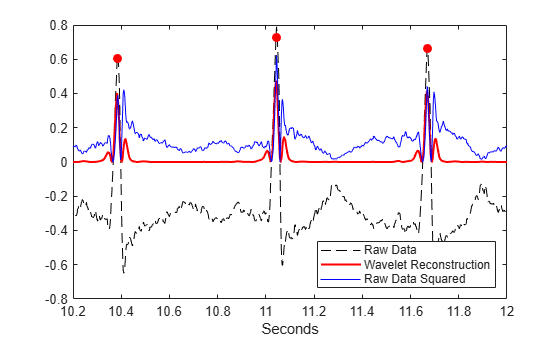# Real-time wavelet decomposition and reconstruction for ECG feature extraction

I need to locate R-peaks in an ECG signal. I'm using wavelets to extract QRS complexes:
First, I decompose the signal using a maximal overlap discrete wavelet transform with the Symlet 4 wavelet. This wavelet resembles the QRS complexes I want to detect. I then copy scales 4 and 5, because this band maximizes QRS energy. The wavelet coefficients on scales 1-3, and the level 5 approximation coefficients are set to zero. Then I perform an inverse wavelet transform on these modified coefficients. The result is a signal in the time domain that contains mostly QRS complexes, and has very high amplitude at the R-peaks.
I just use a peak detector on this signal to locate the R-peaks.Everything is well-explained here: https://mathworks.com/help/wavelet/ug/r-wave-detection-in-the-ecg.html

This is the code I use:

wt = modwt(rawECGsignal_buffer,5);
wtrec = zeros(size(wt));
wtrec(4:5,:) = wt(4:5,:);
filtered = imodwt(wtrec,'sym4');


From what I understand, the Symlet 4 is a biorthogonal wavelet, which means that the decomposition and reconstruction can be implemented as a bank of high- and low-pass FIR filters.Right now, I read the live ECG data into a 5 second buffer, and then perform the wavelet filter on the buffer (as described above). This seems rather inefficient, because I have to filter the entire buffer again when new data comes in.
Is it possible to implement this in a way that allows for real-time filtering of an ECG signal without buffering?
(I.e. single value in → wavelet 'filter' → single filtered/reconstructed value out)

Right now, I read the live ECG data into a 5 second buffer, and then perform the wavelet filter on the buffer (as described above). This seems rather inefficient, because I have to filter the entire buffer again when new data comes in.

You cannot avoid the filtering / reconstruction steps over the buffer with this kind of processing.

Is it possible to implement this in a way that allows for real-time filtering of an ECG signal without buffering? (I.e. single value in → wavelet 'filter' → single filtered/reconstructed value out)

Yes.

For block processing, you can use the overlap add or overlap save methods which can also work straight in the time domain.

For sample-by-sample processing, you can start with the basic formula of discrete convolution:

$$y[n] = \sum_{k=0}^{k<N} x[n-k] \cdot h[k]$$

Where $y$ is the output, $x$ is the input and $h$ is the impulse response, or "filter coefficients".

To get rid of the indexing by $n$, shift the input $x$ by one sample and place the newly acquired sample in the beginning of $x$. You can do this in MATLAB with circshift. So, this would probablly look like x=circshift(x,1);x=input_value.

This now leaves us with getting the $h$ coefficients. You can get those for the wavelets you are using, in MATLAB, using wfilters.

This would cover the application of 1 filter. You will have to repeatedly do this for all the levels you need for both the directions of analysis and reconstruction plus the steps of decimation / upsampling.

So, overall, denoising the ECG in such a way is very demanding. Were the typical QRS detectors (possibly with the addition of "pulse-filters") failing to provide a robust enough R-R sequence?

Hope this helps.

• Thanks a lot for your reply. I don't really see how the filter bank can be implemented. I understand how a single real-time FIR filter works, and how to find the coefficients, but I don't understand how you would cascade them together, and the down/up sampling confuses me. (I understand the diagram, but I don't know how to implement this in real-time.) Dec 11, 2017 at 19:34
• @tttapa I got a lot of good understanding out of this article: A really friendly guide to wavelets. It describes how a wavelet transform can be implemented as a filter bank. Feb 17, 2021 at 23:02